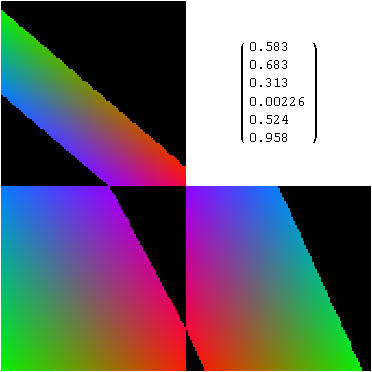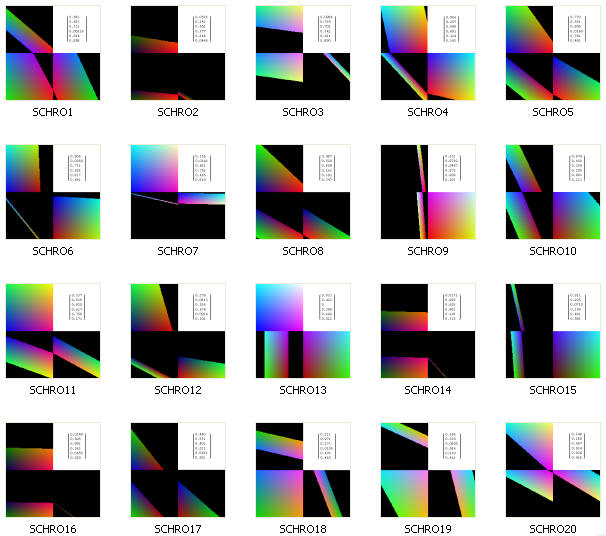Example 5. Random Planes Passing Through a Schrödinger Cube

Here, we look from three different views into the inside of a transparent Schrödinger cube, wherein x, y, z are R, G, B, respectively, and a randomly oriented plane intersects the solid cube's colors.  All six variables (x, y, z, R, G, B) are on exactly the same scale from 0 to 1, such that a position in 3D space determines a color and vice versa.  A plane intersecting the cube generates  a set of coplanar colors that can be viewed from various directions (5.1).One can think of this image as a 3-D cube by folding the lower right panel to the left, projecting its right edge out of the page, and then folding those two planes up over the upper left panel.  This folding produces 3 sides of the six-sided cube. The upper left panel is the 'bottom' view (x, y plane) of the color cube.  Its four corners show the superposition of the cube's eight corners into four flattened 2-D corners: C/G, W/Y, M/R, B/K (starting from the upper left, then clock-wise).  The lower left panel is the front view of the cube (x, z plane), with color corners B/C, M/W, R/Y, K/G superimposed in this projection from 3D to 2D.  Finally, the lower right panel shows the right side of the color cube (y, z  plane), with corners M/B, W/C, Y/G, R/K (clock-wise from upper left corner). These are the same color code abbreviations and axis orientations used in Example 2's schematic of the Schrödinger color cube.  Upper right panel:  In matrix form (top to bottom), the first three values represent the position of a point within the cube at (x, y, z) , and the second three values give a normal vector of a plane passing through this specific point.  A unique point and normal vector fully describe a plane as their dot product must equal zero.  As the six values in the upper right panel are randomized, other randomly oriented planes are generated that intersect at least one point in the Schrödinger cube.  Below, 20 frames are shown (5.2) as this process is repeatedly randomized and redrawn..It is interesting to note that any one of these images can be transmitted to a recipient by a string of six numbers that specify a point and a normal vector.  One only needs to know the layout of the cube, i.e.,  x, y, z is R, G, B.  That is an image compression factor of approximately 100 x 100 x (8+8+8) = 240,000 to 6. As larger images are used (e.g., 1,000 x 1,000), the first number increases (e.g., 24 million) but the second number (6) does not.  Surprisingly, the image compression factor gets better as the images get larger.  This has possible applications in the transmission of large images through a narrow bandwidth.

Here is a PDF copy of the Mathematica source code for Example 5, and here is the corresponding Mathematica .nb .